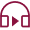Stock / Share Market
Last Updated: 2022-09-21T15:14:27+05:30## How to Calculate Dividend per Share

If you are considering buying a company's shares, you should know how investing in shares can help you make money. There are two ways to make a profit from shares. First, as time goes on, the shares' value increases. That is your first way of income from investing in shares.

The second way that shares make money is through dividends, which companies pay to their shareholders quarterly or yearly. Let's look at a dividend per share, how to calculate dividends per share, some examples, etc.

## What is Dividend Per Share (DPS)?

The amount of money a company distributes to its shareholders in the form of dividends for each share is referred to as the dividends per share.
Now, you might be wondering How to find the dividend per share?
First, divide the total amount of dividends paid out by the total number of outstanding shares.

It is easy to evaluate an investor's investment return mainly by looking at the dividends paid out each share. It is frequently used in calculations to determine a stock's value and evaluate the relative worth of the shares of other companies.

## Dividend Per Share Formula & calculation

Dividend per share formula-

Dividend per share - Dividend/Number of shares

If you receive any one-time dividend payments during an irregular payment cycle period, you will deduct that sum from the total dividends paid:

Dividend per share (DPS) - Dividend-one-time dividend/Number of shares

There are two different ways that you can utilize to answer the question of how to find dividends for each share. The method you employ is going to be determined by the information that is easily accessible to you.

## Dividend Method

The quickest and least complicated option is the common dividend per share formula.

Earnings per Share (EPS) Method

The earnings per share (EPS) approach demands that you know the firm's net income and use it to calculate EPS and the dividend payout ratio first. You cannot use this method if you do not see the company's net income.
No matter which of these two approaches you choose, the dividend paid for each share ought to stay the same.

## Types of Dividends

Dividends are typically paid out in cash to investors, but that isn't always the case. There are many different kinds of dividends, including the following:

Property Dividend

The company distributes the dividend as an asset, which may include property, plant, equipment, a car, inventory, and other similar things.

Liquidating Dividends

The company or business sells all of its assets and then distributes the proceeds to its shareholders as dividends. When a company is set to go out of business, liquidating dividends are typically paid to shareholders.

Cash Dividends

This is the most regular dividend that shareholders get paid out on each share they own. It is merely a monetary payment, and the value may be determined using any of the two methods presented earlier.

Scrip Dividends
The company has promised stockholders that they will be paid at a later time. Scrip dividends can be considered a promissory note that promises to pay shareholders at some point in the future.

## Calculating DPS from the Income Statement

When a company maintains a stable dividend payment ratio, using the income statement, one can arrive at a rough estimate of the company's dividend per share. To know  how to get dividend per share using the income statement, the following steps need to be taken:

1.  Know the company's net profit - The income statement will typically conclude by presenting the net income at the bottom.

2.  Find out how many shares are outstanding - The number of outstanding shares may generally be found on the company's balance sheet. If there are treasury shares, deduct that number from the total number of issued shares to obtain the number of outstanding shares.

3.  Divide the net income by the total number of outstanding shares - The earnings per share can be calculated by taking the net income and dividing it by the total number of shares outstanding (EPS).

4.  Find out what the average payout ratio is for the company - You can estimate the average payout ratio by looking at dividend payments made in the past.

5.    The dividend per share - can be calculated by multiplying the payout ratio by the net income per share.

## Sample Dividend per Share Calculation

For instance, firm A has distributed yearly dividends totaling Rs. 20,000 over the past years. Outstanding shares at the beginning of the time period were 4000, and impressive shares at the end were 7000.

Let's go ahead and know how to calculate dividends per share for company A.

In this case, we can use a simple average to find the average number of outstanding shares.

•    The number of shares outstanding at the starting was 4,000; at the conclusion, it was 7,000.

•    Using the simple average, we can calculate the average number of shares that are now outstanding as follows: = (4000 + 7000) / 2 = 11,000 / 2 = 5500.

•    The total amount of dividends paid each year was Rs20,000.00.

By applying the DPS formula, we get-
Dividend per share -  Dividend / Number of shares    =        Rs. 20,000 / 5500
= Rs. 3.64 per share

## Key Takeaways

A dividend per share is a basic yet insightful financial ratio that can be used to analyze a company's success. Investors and the company's management might both benefit from using it.

Knowing how to get dividends per share can serve as a source of income for investors, and reinvesting them instead of spending them can boost their long-term compounded returns. It makes it easier for investors to engage in tax planning of their dividend income.

Resend OTP